# Weyl theorem

(diff) ← Older revision | Latest revision (diff) | Newer revision → (diff)
The spectrumof a (possibly unbounded) self-adjoint operatoris naturally divided into a point spectrum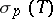(i.e., the set of eigenvalues) and a continuous spectrum, both subsets of the real axis (cf. also Spectrum of an operator). The continuous spectrum is very sensitive against perturbations. The Weyl–von Neumann theorem [a2], Sect. X.2.2, states that for any self-adjoint operatorin a separable Hilbert space there exists a (compact) self-adjoint operatorin the Hilbert–Schmidt class (cf. also Hilbert–Schmidt operator) such that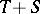has a pure point spectrum. Moreover,can be chosen arbitrarily small in the Hilbert–Schmidt norm. The set of eigenvalues obtained by perturbation of a continuous spectrum is dense in any interval covered by the continuous spectrum of the unperturbed operator. In spectral theory one introduces therefore another subset of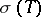which is more stable under perturbations. The essential spectrum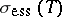is defined as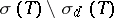, whereis the set of all isolated eigenvalues with finite multiplicity. H. Weyl proved (in a special case) the following result [a4], which is now commonly known as Weyl's theorem: Letbe self-adjoint andsymmetric and compact. Then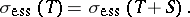In fact, it is even sufficient to require the relative compactness of, which means that the operatoris compact. Another variant of the Weyl theorem states that two self-adjoint operatorsandhave the same essential spectrum if the difference of the resolventsis compact [a3] (cf. also Resolvent). The Weyl theorem is very important in mathematical quantum mechanics, where it serves to prove thatfor a large class of two-body potentials. (A generalization to the-body problem is the HVZ theorem by W. Hunziker, C. van Winter, and G. Zhislin, see [a3].) The situation is considerably more complicated for non-self-adjoint operators, where there are several possible definitions of the essential spectrum [a1]. In general, one defines the Weyl spectrum as the largest subset of the spectrum that is invariant under compact perturbations.